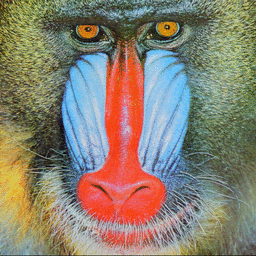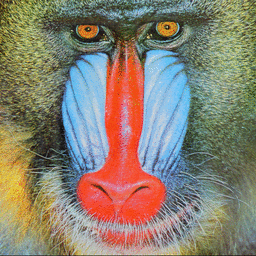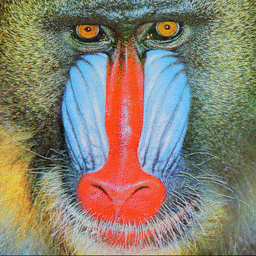## PAGECURL

 Applies a pagecurl effect to the lower right corner of an image.

### EXAMPLES

 Variation In Shading Mode With Ellipticity=0 (circle) Original ImageArguments: -m plain Arguments: -m grad Arguments: -m doublegrad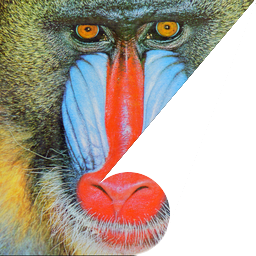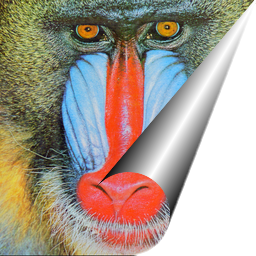Variation In Shading Mode With Color With Ellipticity=0 (circle) Original ImageArguments: -m plain -c wheat Arguments: -m grad -c wheat Arguments: -m doublegrad -c wheat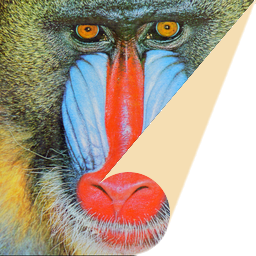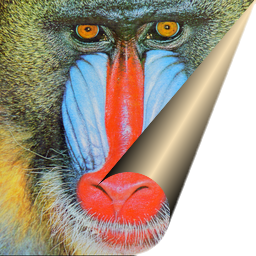Variation In Shading Mode With Ellipticity=0.5 (ellipse) Original ImageArguments: -m plain -e 0.5 Arguments: -m grad -e 0.5 Arguments: -m doublegrad -e 0.5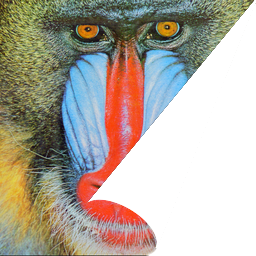Variation In Amount With Mode=grad AND Ellipticity=0 (circle) Original ImageArguments: -a 20 -m grad Arguments: -a 50 -m grad Arguments: -a 80 -m gradVariation In Amount With Mode=grad AND Ellipticity=0.5 (ellipse) Original ImageArguments: -a 20 -m grad -e 0.5 Arguments: -a 50 -m grad -e 0.5 Arguments: -a 80 -m grad -e 0.5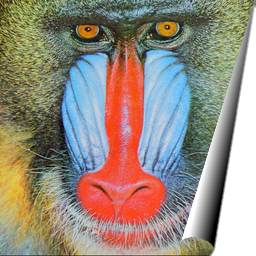Variation In Contrast For Mode=grad With Ellipticity=0 (cirlce) Original ImageArguments: -m grad -g 10 Arguments: -m grad -g 15 Arguments: -m grad -g 20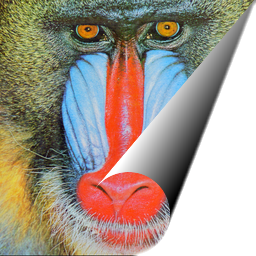Variation In Contrast For Mode=doublegrad With Ellipticity=0 (cirlce) Original ImageArguments: -m grad -d -15 Arguments: -m grad -d 0 Arguments: -m grad -d 15Variation In Apex Location For Mode=grad And Ellipticity=0 (circle) Original ImageArguments: -m grad -y 100 -b green Arguments: -m grad -x 220 -b green Arguments: -m grad -x 255 -y -100 -b green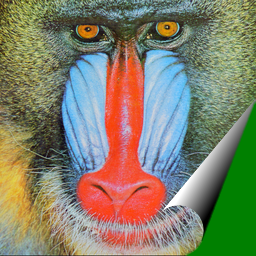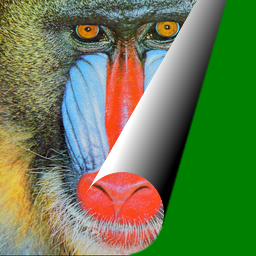Animations (scripts modified from one provided by Anthony Thyssen) Original Imageimage="mandril3.png" for ((i=5; i<=105; i=i+10)); do echo >&2 i=\$i pagecurl -a \$i "\$image" pam: done | convert \ -dispose background \ -delay 100 "\$image" \ -delay 10 - \ \( -delay 10 -clone -1-1 \) \ -delay 100 "\$image" \ -loop 0 -layers Optimize \ pagecurl_animation1.gif image="mandril3.png" bgimage="zelda1.jpg" for ((i=5; i<=105; i=i+10)); do echo >&2 i=\$i pagecurl -a \$i "\$image" "\$bgimage" pam: done | convert \ -dispose background \ -delay 100 "\$image" \ -delay 10 - \ \( -delay 10 -clone -1-1 \) \ -delay 100 "\$image" \ -loop 0 -layers Optimize \ pagecurl_animation2.gif image="mandril3.png" bgimage="zelda1.jpg" width=`identify -format '%[fx:w]' "\$image"` height=`identify -format '%[fx:h]' "\$image"` start=\$((width+height/2)) start1=\$((start-start/20)) for ((i=\$start1; i>=0; i=i-\$((start/20)))); do x=\$i; y=\$((i-width)) echo >&2 i=\$i x=\$x y=\$y pagecurl -x \$x -y \$y "\$image" "\$bgimage" pam: done | convert \ -dispose background \ -delay 100 "\$image" \ -delay 10 - \ \( -clone -1-1 \) \ -delay 100 "\$image" \ -loop 0 -layers Optimize \ pagecurl_animation3.gif